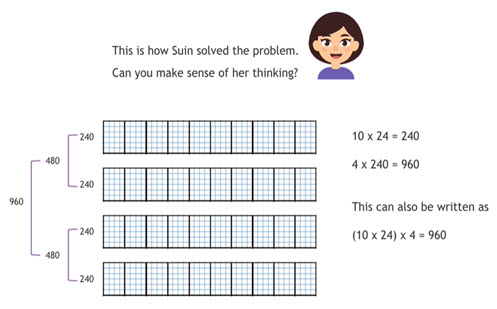Go to website

# reSolve bakery 3

Models and compares different multiplication strategies for solving the 'The cupcake order' problem. It then launches the 'Cupcake boxes' problem, which draws on various concepts including two-digit multiplication, shape and area.

Year level(s) Year 5
Audience Student, Teacher
Purpose Teaching resource, Teaching strategies
Format Video
Teaching strategies and pedagogical approaches Questioning, Concrete Representational Abstract model, Targeted teaching, Worked examples
Keywords strategy, partitioning, multiplication, renaming, doubling, problem solving, reasoning, understanding, fluency, centimetres, area, explicit teaching

## Curriculum alignment

Curriculum connections Numeracy
Strand and focus Number, Build understanding
Topics Multiplication and division, Operating with number
AC: Mathematics (V9.0) content descriptions
AC9M5N06
Solve problems involving multiplication of larger numbers by one- or two-digit numbers, choosing efficient calculation strategies and using digital tools where appropriate; check the reasonableness of answers

AC9M5M02
Solve practical problems involving the perimeter and area of regular and irregular shapes using appropriate metric units

AC9M5N02
Express natural numbers as products of their factors, recognise multiples and determine if one number is divisible by another

Numeracy progression Multiplicative strategies (P6, P7)
Understanding units of measurement (P7)